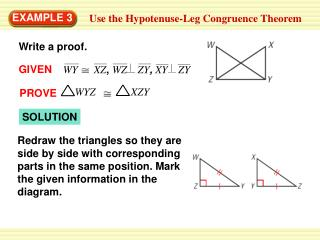DownloadDownload PresentationEXAMPLE 3

# EXAMPLE 3

Télécharger la présentation## EXAMPLE 3

- - - - - - - - - - - - - - - - - - - - - - - - - - - E N D - - - - - - - - - - - - - - - - - - - - - - - - - - -
##### Presentation Transcript

1. GIVEN WY XZ,WZ ZY, XY ZY WYZXZY PROVE Redraw the triangles so they are side by side with corresponding parts in the same position. Mark the given information in the diagram. EXAMPLE 3 Use the Hypotenuse-Leg Congruence Theorem Write a proof. SOLUTION

2. STATEMENTS REASONS H WY XZ Given WZ ZY, XY ZY Given Definition of lines Z andY are right angles Definition of a right triangle WYZand XZY are right triangles. ZY YZ L Reflexive Property of Congruence WYZXZY HL Congruence Theorem EXAMPLE 3 Use the Hypotenuse-Leg Congruence Theorem

3. EXAMPLE 4 Choose a postulate or theorem Sign Making You are making a canvas sign to hang on the triangular wall over the door to the barn shown in the picture. You think you can use two identical triangular sheets of canvas. You knowthatRP QS andPQ PS . What postulate or theorem can you use to conclude that PQR PSR?

4. You are given that PQ PS. By the Reflexive Property, RP RP. By the definition of perpendicular lines, both RPQ and RPSare right angles, so they are congruent. So, two sides and their included angle are congruent. ANSWER You can use the SAS Congruence Postulate to conclude that . PQR PSR EXAMPLE 4 Choose a postulate or theorem SOLUTION

5. Redraw ACBand DBCside by side with corresponding parts in the same position. for Examples 3 and 4 GUIDED PRACTICE Use the diagram at the right.

6. STATEMENTS REASONS H AB DC Given AC BC, DB BC Given Definition of lines C B Definition of a right triangle ACBand DBC are right triangles. for Examples 3 and 4 GUIDED PRACTICE Use the diagram at the right. Use the information in the diagram to prove that ACB DBC

7. BC CB L Reflexive Property of Congruence ACBDBC HL Congruence Theorem for Examples 3 and 4 GUIDED PRACTICE STATEMENTS REASONS